## Pages

### Teaching Linear EquationsI love algebra. Geometry teachers may disagree that it's the best math, and my own algebra 2 heart aches a little at this confession, but there are just so many amazing things about it. Algebra is the first time students are introduced to all forms of a linear equation. It's the first time kids really need to write things down. Kids who have struggled with math, tediously writing everything down throughout elementary and middle school, will finally see their hard work pay off in algebra when keeping track of steps matters. And then there's the direct connection between algebra and calculus. We even use the point-slope form of a linear equation to find the equations of tangent lines.

In this post, I want to share some of the linear equations activities I've made over the years and their recent digital updates.

Linear equations are really first introduced in 7th grade along with constant of proportionality and unit rates. Linear equations are one of those topics that just keep coming around in different forms, and can often give students a little trouble. I made a set of 3 interactive notebook flippables for the slope formula, the slope-intercept equation and the point-slope form of a linear equation so that students always have a handy reference for the linear equations they will see in class.

Interactive notebooks weren't a thing when I was a kid, but I would have been 1000% into them if they had been.

Here is how the slope-intercept form flippable goes together. All pieces are on one sheet of paper that gets cut, stacked and stapled. The slope and point-slope flippables go together the same way. The flippables can be glued into a notebook or can stand alone as handy references.

Under each flap of each flippable is an explanation of each variable. After a few teacher requests, I updated the linear equations flippables file with a digital version in Google Slides.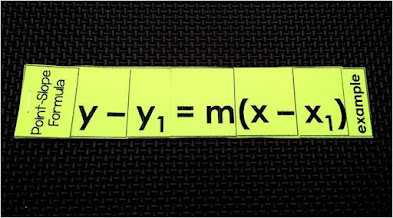Here is a closeup of the point-slope flippable.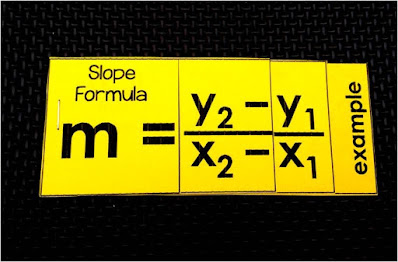And here is the slope formula flippable.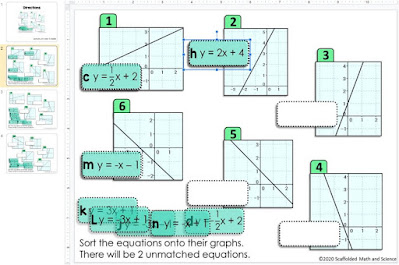Linear equations sorting activity - digital version

I added a digital version in Google Slides to this linear equations sorting activity. All equations are in slope-intercept form. The activity helps reinforce the skill of identifying slope and y-intercept in linear equations.

Here is a closeup of puzzle #2. Students identify the equations of all 4 lines, then type the 4-letter code to unlock the puzzle. There are 5 puzzles in all. This engaging digital activity is an answer-validated Google Form, offering the immediate feedback to students that they love.
My friend Alex from Middle School Math Man and I have been working on a new game series called Voyage to the Treasure! This one above is a Voyage to the Treasure! writing linear equations game. In this game, students are on the same team, working their way to the treasure before Math Monster.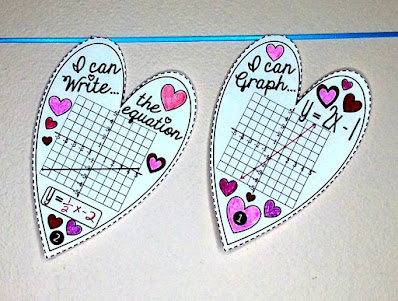Linear equations hearts
This slope-intercept hearts pennant is specially designed for love-fueled Valentine's Day when there are many more googly eyes than assignments getting done. Students can color a little as they graph and identity their linear equations.Linear equations ornaments
I have made a bunch of holiday math pennants as a way for students to do math, stay engaged and decorate their classrooms for the holidays. Above is a set of linear equations ornaments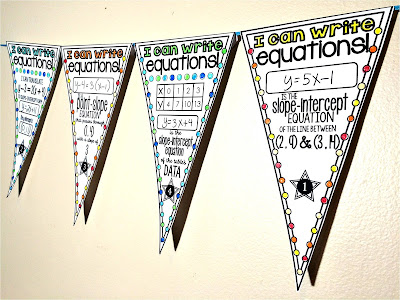Linear equations pennant

Because sometimes linear equations hit during other times of year, I made this linear equations pennant that covers writing equations given different pieces of information, like tables and points.Linear equations puzzle

This linear equations puzzle - print and digital asks students to match 3 forms of linear equations to their graphs and is a great team activity. The digital version is in Google Slides.linear equations reference - algebra word wall

While teaching algebra 2, I found myself drawing linear equations on the board over and over again to make links between y-intercepts, zeros, etc. from linear graphs and the nonlinear functions we were graphing. That summer, I decided to give linear equations a permanent home in my classroom and started work on an algebra word wall. Since then it has grown a lot and now also includes a clickable digital version in Google Slides.Linear equations cheat sheet

For some reason, our linear equations review unit was always the biggest struggle in algebra 2. I made this linear equations cheat sheet for students who are struggling to grasp graphing lines. It focuses on creating tables from the y-intercept and one other coordinate, then graphing from the table. The sheet can be slipped into a notebook or enlarged to make an anchor chart for graphing from a table.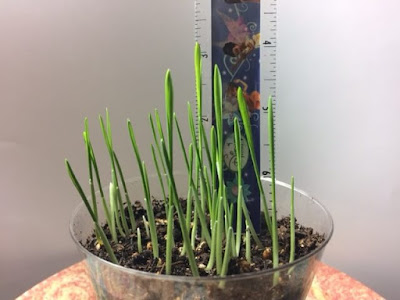Linear equations project

Back when I had taught algebra 1, one of my favorites parts was a graphing grass linear equations project. The students got so into it and the project tied to so many different parts of our curriculum. Collecting data, plotting coordinates, finding slope, finding line of fit, writing the equations of lines, extrapolation, and even piecewise functions when students gave their grass a "haircut". Students got so attached to their little cups of grass and we even had some great ecology discussions. You can read more about the project in the post Graphing Grass Linear Equations Project.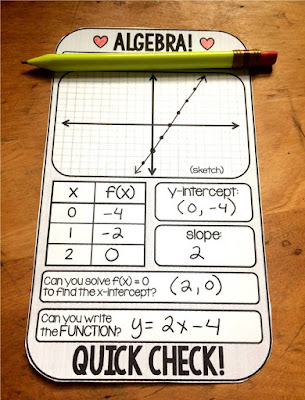Linear equations template
Warm ups, do nows, bell ringers... whatever you call them they are so important. Warm up templates have changed my teaching almost as much as word walls have. This Algebra warm up template makes practicing graphing linear equations, completing tables, finding slope, finding y-intercepts, finding x-intercepts and writing equations given a graph (this one is always the hardest, even for my Algebra 2 students) super quick and easy. And the best part? Print a stack and you're good to go for a while. You can find the free linear equations template here.Algebra Activities Bundle w/ digital updates

This big algebra activities bundle will help you differentiate your algebra 1 curriculum and engage your students. From my experience as both a mainstream and special education math teacher, I know that all students need engaging, differentiated math activities that hit the standards while also being fun! This is my goal for every activity I create and what is inside this algebra activities bundle.

1.